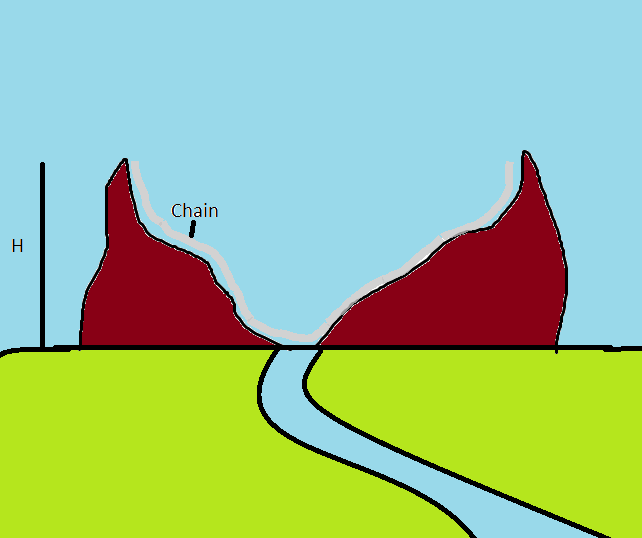# Chain on a hillockConsider two hillocks adjacent to each other with smooth surfaces,

They may have any shape, A silver chain lying midway between them initially has "half" of its length on one hillock and half on the other hillock ,, and rises to an equal height 'H" initially on either of them, (Refer to figure)

The earth as usual has gravitational field.

And the slope of the part where the chains end terminate have slope 90 degree.

You may refer to the figure now

Now if i slightly displace the chain towards the centre and release, find the time period of oscillations.

( in seconds)

DETAILS

$\bullet$ Mass= 1

$\bullet$ height =1

$\bullet$ l= 1

$\bullet$ g =10

NOTE

$\bullet$ Assume all ideal Conditions.

HINT

$\bullet$ internal tension of chain cancels out

$\bullet$ It is a free chain.

×